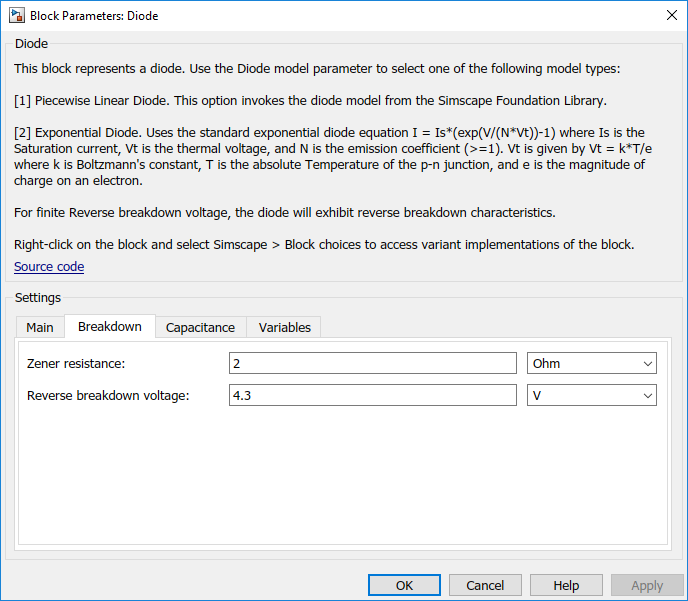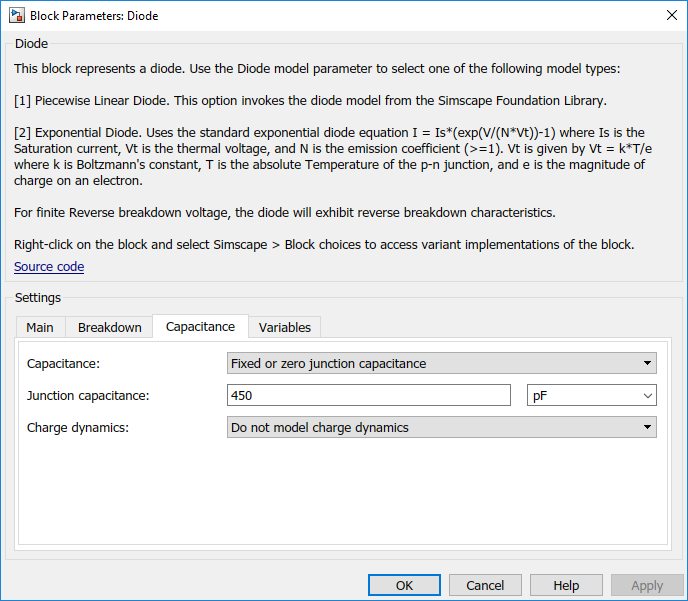## Parameterize a Piecewise Linear Diode Model from a Datasheet

The Triangle Wave Generator example model, also described in Build and Simulate a Simple Circuit, contains two zener diodes that regulate the maximum output voltage from an op-amp amplifier circuit. Each of these diodes is implemented with the Simscape™ Electrical™ Diode block, parameterized using the ```Piecewise Linear``` option. This simple model is sufficient to check correct operation of the circuit, and requires fewer parameters than the `Exponential` option of the Diode block. However, when specifying the parameters, you need to take into account the bias condition that will be used in the circuit. This example explains how to do this.

The Phillips Semiconductors datasheet for a BZX384–B4V3 gives the following data:

 Working voltage, VZ(V) at IZtest = 5 mA 4.3 Diode capacitance, Cd(pF) 450 Reverse current, IR(μA) at VR = 1 V 3 Forward voltage, VF(V) at IF = 5 mA 0.7

In the datasheet, the tabulated values for VF are for higher forward currents. This value of 0.7V at 5mA is extracted from the datasheet current-voltage curve, and is chosen as it matches the zener current used when quoting the working voltage of 4.3V.

To match the datasheet values, the example sets the piecewise linear diode block parameters as follows:

• Forward voltage. Leave as default value of 0.6V. This is a typical value for most diodes, and the exact value is not critical. However, it is important that the value set is taken into account when calculating the On resistance parameter.

• On resistance. This is set using the datasheet information that the forward voltage is 0.7V when the current is 5mA. The voltage to be dropped by the On resistance parameter is 0.7V minus the Forward voltage parameter, that is 0.1V. Hence the On resistance is 0.1V / 5mA = 20 Ω.

• Off conductance. This is set using the datasheet information on reverse current. The reverse current is 3μA for a reverse voltage of 1V. Hence the Off conductance should be set to 3μA / 1V = 3e-6 S.

• Reverse breakdown voltage. This parameter should be set to the datasheet working voltage parameter, 4.3V.

• Zener resistance. This needs to be set to a suitable small number. Too small, and the voltage-current relationship becomes very steep, and simulation convergence may not be as efficient. Too large, and the zener voltage will be incorrect. For the Diode block to be representative of the real device, the simulated reverse voltage should be close to 4.3V at 5mA (the reverse bias current provided by the circuit). Allowing a 0.01 V error on the zener voltage at 5mA, the zener resistance RZ will be 0.01V / 5mA = 2 Ω.

• Junction capacitance. This parameter is set to the datasheet diode capacitance value, 450 pF.